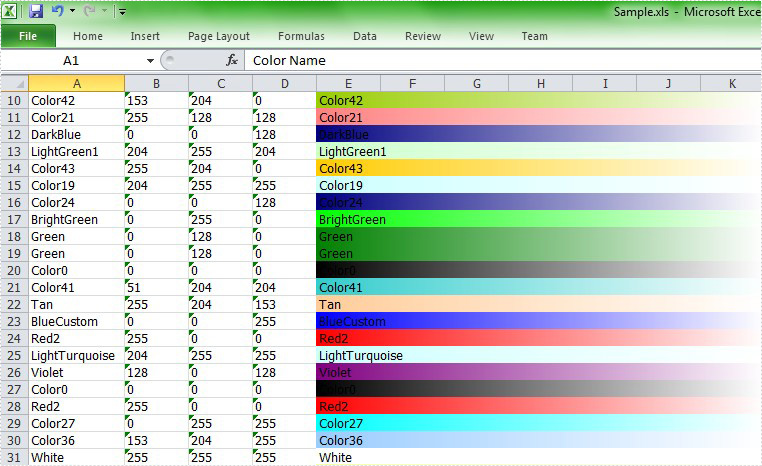# Insert Interior in Excel in C#, VB.NET

### What is Excel Interior?

Excel provides essentially no support in worksheet functions for Working with cell colors. However, colors are often used in spreadsheets to indicate some sorts of value or category. Thus comes the need for functions that can work with colors on the worksheet. So it appears in the version in Excel 2007 as a new function. It contains all kinds of colors. Below I will show you how to insert interior in Excel with MS Excel and how to do this with Spire.XLS.

### How to insert interior in Excel with MS Excel?

To insert interior in Excel with Microsoft Excel, you can follow the sections below:

• Open the worksheet in Excel
• Highlight the zones that you want to insert interior
• Rightclick and choose Setting Cell Format
• Choose Fill->Fill Effect in the dialog box of Setting Cell Format
• In the box, you can change the Color and the Shade Format to your desired effect

### How to Insert Interior with Spire.XLS?

It's convenient to realize C#/.NET Excel Integration via Spire.XLS. In interior method, to realize interior you may set the color gradient by assigning sheet.Range[string.Format("E{0}:K{0}", i)].Style.Interior.FillPattern property with ExcelPatternType.Gradient. You can set the BackKnownColor and ForeKnownColor of the sheet. What's more, you can set the gradient style, in the demo, we set the gradient style vertical. In order to reflect the effect, we merge the worksheet range from E to K. In this demo, we use Enum method to enumerate many kinds of colors and define a random object to fill the cell with a gradient color randomly.

First, let's preview the effect screenshot:Here comes to the full code in C# and VB.NET.

[C#]
```using Spire.Xls;
using System.Drawing;
using System;

namespace Interior
{
class Program
{
static void Main(string[] args)
{
//Create a workbook
Workbook workbook = new Workbook();

//Initialize the worksheet
Worksheet sheet = workbook.Worksheets;

//Specify the version
workbook.Version = ExcelVersion.Version2007;

//Define the number of the colors
int maxColor = Enum.GetValues(typeof(ExcelColors)).Length;

//Create a random object
Random random = new Random((int)System.DateTime.Now.Ticks);

for (int i = 2; i < 40; i++)
{
//Random backKnownColor
ExcelColors backKnownColor = (ExcelColors)(random.Next(1, maxColor / 2));
sheet.Range["A1"].Text = "Color Name";
sheet.Range["B1"].Text = "Red";
sheet.Range["C1"].Text = "Green";
sheet.Range["D1"].Text = "Blue";

//Merge the sheet"E1-K1"
sheet.Range["E1:K1"].Merge();
sheet.Range["A1:K1"].Style.Font.IsBold = true;
sheet.Range["A1:K1"].Style.Font.Size = 11;

//Set the text of color in sheetA-sheetD
string colorName = backKnownColor.ToString();
sheet.Range[string.Format("A{0}", i)].Text = colorName;
sheet.Range[string.Format("B{0}", i)].Text = workbook.GetPaletteColor(backKnownColor).R.ToString();
sheet.Range[string.Format("C{0}", i)].Text = workbook.GetPaletteColor(backKnownColor).G.ToString();
sheet.Range[string.Format("D{0}", i)].Text = workbook.GetPaletteColor(backKnownColor).B.ToString();

//Merge the sheets
sheet.Range[string.Format("E{0}:K{0}", i)].Merge();

//Set the text of sheetE-sheetK
sheet.Range[string.Format("E{0}:K{0}", i)].Text = colorName;

//Set the interior of the color
}

//AutoFit Column
sheet.AutoFitColumn(1);

//Save the file
workbook.SaveToFile("Sample.xls",ExcelVersion.Version97to2003);

//Launch the file
System.Diagnostics.Process.Start("Sample.xls");
}
}
}
```
[VB.NET]
```Imports Spire.Xls
Imports System.Drawing
Imports System

Module Module1

Sub Main()
'Create a workbook
Dim workbook As New Workbook()

'Initialize the worksheet
Dim sheet As Worksheet = workbook.Worksheets(0)

'Specify the version
workbook.Version = ExcelVersion.Version2007

'Define the number of the colors
Dim maxColor As Integer = [Enum].GetValues(GetType(ExcelColors)).Length

'Create a random object
Dim random As New Random()

For i As Integer = 2 To 39
'Random backKnownColor
Dim backKnownColor As ExcelColors = DirectCast(random.[Next](1, maxColor \ 2), ExcelColors)
sheet.Range("A1").Text = "Color Name"
sheet.Range("B1").Text = "Red"
sheet.Range("C1").Text = "Green"
sheet.Range("D1").Text = "Blue"

'Merge the sheet"E1-K1"
sheet.Range("E1:K1").Merge()
sheet.Range("A1:K1").Style.Font.IsBold = True
sheet.Range("A1:K1").Style.Font.Size = 11

'Set the text of color in sheetA-sheetD
Dim colorName As String = backKnownColor.ToString()
sheet.Range(String.Format("A{0}", i)).Text = colorName
sheet.Range(String.Format("B{0}", i)).Text = workbook.GetPaletteColor(backKnownColor).R.ToString()
sheet.Range(String.Format("C{0}", i)).Text = workbook.GetPaletteColor(backKnownColor).G.ToString()
sheet.Range(String.Format("D{0}", i)).Text = workbook.GetPaletteColor(backKnownColor).B.ToString()

'Merge the sheets
sheet.Range(String.Format("E{0}:K{0}", i)).Merge()

'Set the text of sheetE-sheetK
sheet.Range(String.Format("E{0}:K{0}", i)).Text = colorName

'Set the interior of the color
Next

'AutoFit Column
sheet.AutoFitColumn(1)

'Save doc file.
workbook.SaveToFile("Sample.xls",ExcelVersion.Version97to2003)

'Launching the MS Word file.
System.Diagnostics.Process.Start("Sample.xls")
End Sub
End Module
```

After running the demo, you will find color interior in your 2007 worksheet.